PSABM6WX - Student Facing Task---Acc7.5 Lesson 21 The Slope of a Fitted Line (8.EE.B.6, 8.SP.A.1, 8.SP.A.2, 8.SP.A.3)

Estimate the slope of the line.Part A)

For each scatter plot, decide if there is an association between the two variables, and describe the situation using one of these sentences:

• For these data, as ________________ increases, ________________ tends to increase.
• For these data, as ________________ increases, ________________ tends to decrease.
• For these data, ________________ and ________________ do not appear to be related.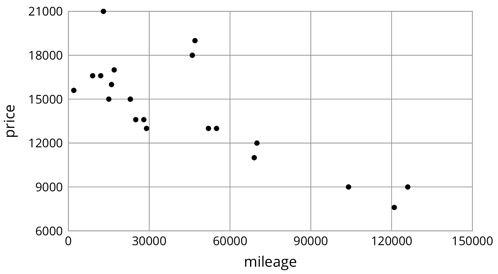Part B)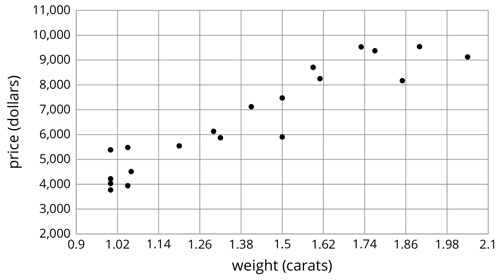Part C)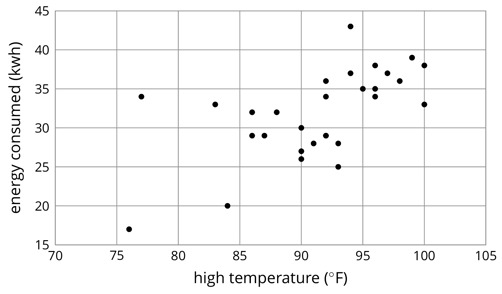Part A)

For each of the situations, a linear model for some data is shown.

1. What is the slope of the line in the scatter plot for each situation?
2. What is the meaning of the slope in that situation?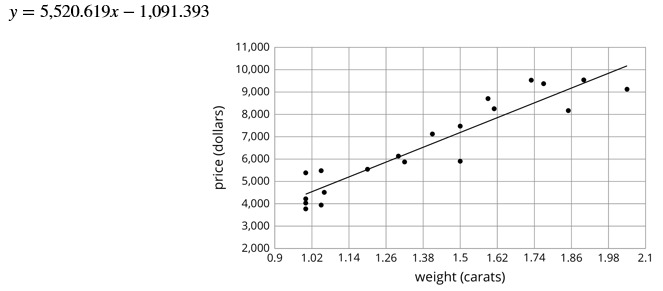slope:

Type your answer below as a number (example: 5, 3.1, 4 1/2, or 3/2):
Part B)

What is the meaning of the slope in this situation?

Part C)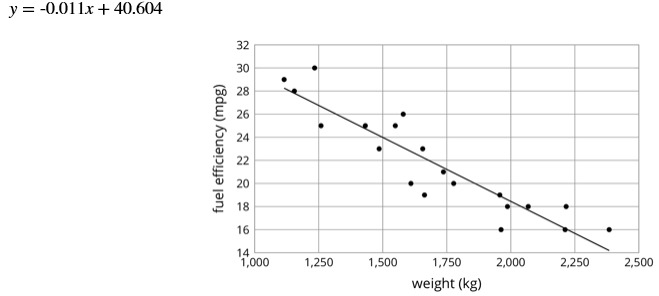slope:

Type your answer below as a number (example: 5, 3.1, 4 1/2, or 3/2):
Part D)

What is the meaning of the slope in this situation?

Part E)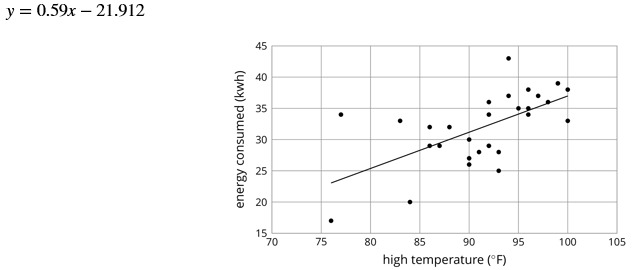slope:

Type your answer below as a number (example: 5, 3.1, 4 1/2, or 3/2):
Part F)

What is the meaning of the slope in this situation?

Part A)

The scatter plot shows the weight and fuel efficiency data used in an earlier lesson along with a linear model represented by the equation y=-0.0114x+41.3021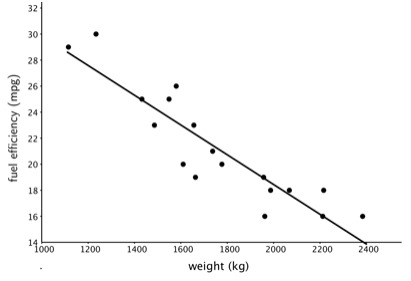What is the value of the slope?

Type your answer below as a number (example: 5, 3.1, 4 1/2, or 3/2):
Part B)

What does the slope mean in this context?

Part C)

What does the other number in the equation represent on the graph? What does it mean in context?

Part D)

Use the equation to predict the fuel efficiency of a car that weighs 100 kilograms.

Type your answer below as a number (example: 5, 3.1, 4 1/2, or 3/2):
Part E)

Use the equation to predict the weight of a car that has a fuel efficiency of 22 mpg.

Type your answer below as a number (example: 5, 3.1, 4 1/2, or 3/2):
Part F)

Which of these two predictions probably fits reality better? Explain.

Part A)

For each of the scatter plots, decide whether it makes sense to fit a linear model to the data.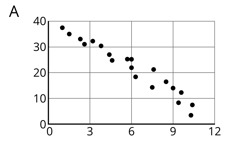Select one:
Part B)

Would the graph of the model have a positive slope, negative slope, or a slope of zero?

Select one:
Part C)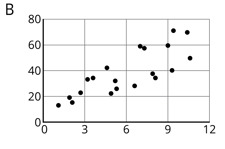Select one:
Part D)

Would the graph of the model have a positive slope, a negative slope, or a slope of zero?

Select one:
Part E)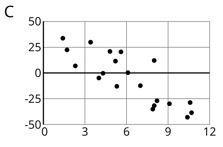Select one:
Part F)

Would the graph of the model have a positive slope, a negative slope, or a slope of zero?

Select one:
Part G)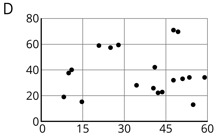Select one:
Part H)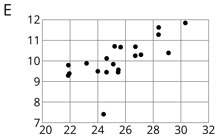Select one:
Part I)

Would the graph of the model have a positive slope, a negative slope, or a slope of zero?

Select one:
Part J)

Which of these scatter plots show evidence of a positive association between the variables?

Select one:
Part K)

Which of these scatter plots show evidence of a negative association between the variables?

Select one:
Part L)

Which do not appear to show an association?

Select one: